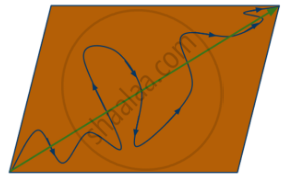Share

# What is the Difference Between Distance Travelled by a Body and Its Displacement ? Explain with the Help of a Diagram. - CBSE Class 9 - Science

ConceptDistance and Displacement

#### Question

What is the difference between distance travelled  by a body and its displacement ? Explain with the
help of a diagram.

#### Solution

 Distance Displacement 1.Distance has only magnitude, with no specified direction. 1.Displacement has magnitude as well as direction. 2.It is a scalar quantity. 2.It is a vector quantity. 3.Two different distances can be added directly. 3.We have to follow the vector addition method to add displacements.

One difference in diagrammatic form is as follows:Here the curved line is the distance traveled and the straight line is the magnitude of the displacement.

Is there an error in this question or solution?

#### Video TutorialsVIEW ALL 

Solution What is the Difference Between Distance Travelled by a Body and Its Displacement ? Explain with the Help of a Diagram. Concept: Distance and Displacement.
S